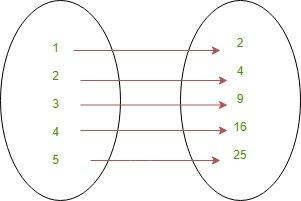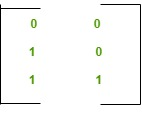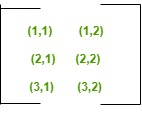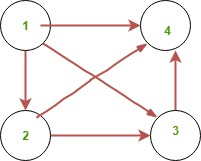# Discrete Mathematics | Representing Relations

Prerequisite – Introduction and types of Relations
Relations are represented using ordered pairs, matrix and digraphs:

1. Ordered Pairs –
In this set of ordered pairs of x and y are used to represent relation. In this corresponding values of x and y are represented using parenthesis.

```Example: {(1, 1), (2, 4), (3, 9), (4, 16), (5, 25)}
This represent square of a number which means if x=1 then y = x*x = 1 and so on.
```2. Representing using Matrix –
In this zero-one is used to represent the relationship that exists between two sets. In this if a element is present then it is represented by 1 else it is represented by 0. In this method it is easy to judge if a relation is reflexive, symmetric or transitive just by looking at the matrix.

```Suppose R is a relation from X={x1, x2, .....xn} to Y={y1, y2....yn}
It is represented by :-
M[i, j]={1, if (Xi, Yj) belongs to R
0, if (Xi, Yj) does not belong to R}
```

If A={1, 2, 3} and B={1, 2} and Relation R is
R = {(2, 1), (3, 1), (3, 2)}
then all corresponding value of Relation will be represented by “1” else “0”.

It is represented as:It’s corresponding possible relations are:3. Digraph –
A digraph is known was directed graph. It consists of set ‘V’ of vertices and with the edges ‘E’. Here E is represented by ordered pair of Vertices.
In the edge (a, b), a is the initial vertex and b is the final vertex.
If edge is (a, a) then this is regarded as loop.

Example: Suppose we have relation forming

`R = {(1, 2), (1, 3), (1, 4), (2, 3), (2, 4), (3, 4)} `

This relation is represented using digraph as: